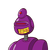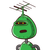# Can anyone plz answer this question. 0.0064 × 10^8​

Can anyone plz answer this question.

0.0064 × 10^8​

### 2 thoughts on “Can anyone plz answer this question. <br /><br />0.0064 × 10^8​”

1.Step-by-step explanation:

=0.0064*10^8

=64*10^(-4)*10^8

=64*10^(-4+8)

=64*10^4

2.640,000 or 6,40,000

Step-by-step explanation:

0.0064 x 10^8

= 64 x 10^4

= 640000.0

Dude if u have to multiply the number with a number which is a multiple of ten

then multiply the number with first digit (which is 1 in this case) and then add the remaining zeros,